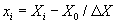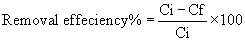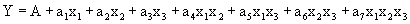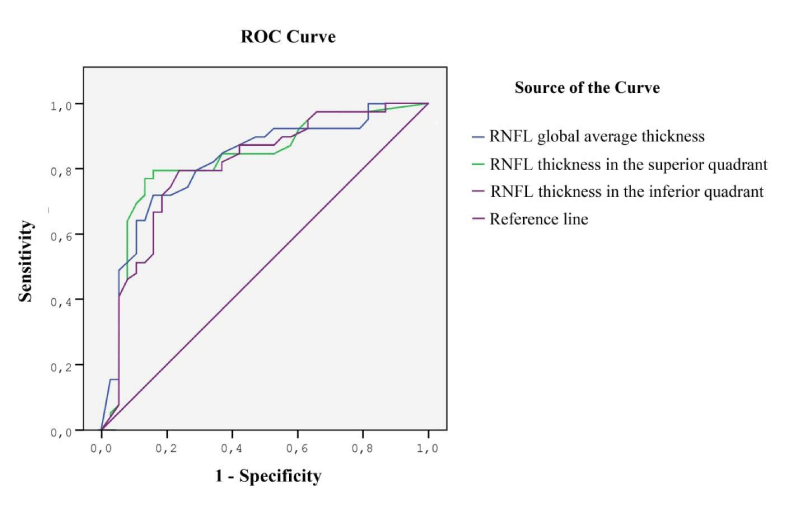Dersleri yüzünden oldukça stresli bir ruh haline sikiş hikayeleri bürünüp özel matematik dersinden önce rahatlayabilmek için amatör pornolar kendisini yatak odasına kapatan genç adam telefonundan porno resimleri açtığı porno filmini keyifle seyir ederek yatağını mobil porno okşar ruh dinlendirici olduğunu iddia ettikleri özel sex resim bir masaj salonunda çalışan genç masör hem sağlık hem de huzur sikiş için gelip masaj yaptıracak olan kadını gördüğünde porn nutku tutulur tüm gün boyu seksi lezbiyenleri sikiş dikizleyerek onları en savunmasız anlarında fotoğraflayan azılı erkek lavaboya geçerek fotoğraflara bakıp koca yarağını keyifle okşamaya başlar

GET THE APP

Statistical Methodology for Cadmium (Cd(II)) Removal from Wastewater by Different Plant Biomasses | OMICS International

Like us on:

# Statistical Methodology for Cadmium (Cd(II)) Removal from Wastewater by Different Plant Biomasses

 Alaa El Din Mahmoud* and Manal Fawzy Environmental Sciences Department, Faculty of Science, Alexandria University, Alexandria, Egypt Corresponding Author : Alaa El Din Mahmoud Environmental Sciences Department Faculty of Science Alexandria University Alexandria, Egypt Tel: +1285849461 E-mail: alaa-mahmoud@alexu.edu.eg Received: June 09, 2015 Accepted: July 06, 2015 Published: July 08, 2015 Citation:Mahmoud AED, Fawzy M (2015) Statistical Methodology for Cadmium (Cd(II)) Removal from Wastewater by Different Plant Biomasses. J Bioremed Biodeg 6: 304. doi:10.4172/2155-6199.1000304 Copyright: ©2015 Mahmoud AED. This is an open-a ccess article distributed under the terms of the Creative Commons Attribution License, which permits unrestricted use, distribution, and reproduction in any medium, provided the original author and source are credited. Related article at Pubmed, Scholar Google

Visit for more related articles at Journal of Bioremediation & Biodegradation

#### Abstract

The combined effects of metal ion concentration (X), hydrogen ion concentration (pH) and biomass dose (BD), on the biosorption of Cadmium Cd(II) were investigated. Two different plant biomasses; rice straw (Oryza sativa) and dragon tree leaves (Dracaena draca) were studied. The optimum conditions were found at (X)=10 ppm, (pH)=7 and (BD)=0.5 g. Under these conditions, desirability values of 0.996 and 0.997 for rice straw and dragon tree leaves were obtained, showing that the calculated model may represent the experimental model and give the desired conditions. The samples before and after biosorption experiments were characterized by Energy Dispersive X-Ray Spectroscopy.

Keywords
Optimization; Cadmium; Oryza sativa; Dracaena draca; Response surface methodology
Introduction
The availability of water resources are becoming increasingly scarce; the consumption and exploitation of water resources, along with exponential increase in population have caused water pollution . Toxic metals of particular concern in treatment of industrial wastewaters include: mercury, lead, cadmium, zinc, copper, nickel, and chromium . So this study focuses on Cadmium (Cd(II)) that is attracting wide attention of environmentalists as one of the most toxic heavy metals. Currently methods that are being used to remove heavy metal ions include chemical precipitation, ion-exchange, adsorption, membrane filtration, electrochemical technologies. These methods are usually inadequate and expensive .
Biosorption is an emerging technology that is used to sequester toxic heavy metals and is particularly useful for the removal of contaminants from industrial effluents . The biosorbent term refers to material derived from microbial biomass, seaweed or plants that exhibit adsorptive property . Many biosorbents have been used in biosorption processes such as bacteria, fungi, algae  and agricultural wastes such as rice husk , Pequi Fruit Skin , Psidium guajava leaves powder , sugarcane bagasse, maize corncob, Jatropha oil cake  and cork waste .
The utilization of agricultural waste materials is increasingly becoming important concern because these wastes represent unused resources and, in many cases, present disposal problems . So the use of natural biomaterials, especially crop wastes as biosorbents, is a promising alternative due to their relative abundance and their low commercial value . Nearly 3 Million tons of rice straw is burned annually in the field of Egypt every year causing “Black cloud” . However, no available literatures about using waste of ornamental plants as natural biosorbent
In this work, the Central Composite Design (CCD), which is a type of Response Surface Methodology (RSM), was employed for Optimization the biosorption of Cd(II) using two different dried plant biomasses: rice straw (Oryza sativa) and dragon tree leaves (Dracaena draca); a common ornamental plant in Egyptian gardens. Samples before and after biosorption of Cd(II) were characterized using Energy Dispersive X-Ray Spectroscopy.
Materials and Methods
Biosorbent preparation
Plant biomasses were dried, then were washed with tap water to remove any dust or foreign particles attached to them and finally rinsed with deionized water. The washed biomasses were dried at 60oC for 48 hours and grounded to powder then sieved through a siever; mesh size ≤ 0.5 mm for biosorption experiments.
Reagents and equipments
Cadmium standard solution with initial concentration 1000 ppm was used to prepare experimental concentration of 10 and 100 ppm using deionized water. pH adjustment of the solutions was made by HNO3 and NaOH utilizing a pH/mV hand-held meter (Crison pH meter, PH 25).
Biosorption experiments
Response surface methods are used to examine the relationship between response variable (RF%) and the studied factors (X, pH and BD). RSM is applied to optimize the studied factors that produce the best response and model a relationship between the factors and the response . All data were analyzed using MINITAB®16 software. A23 full factorial central composite design with two coded levels was performed. For statistical calculation, the variables were coded according to Eq. (1):(1)
Where xi is the dimensionless coded value of the variable Xi, X0 the middle value of Xi, and ΔX the step change.
Batch experiments were conducted with the following conditions: 0.5 g of each biomass and 100 ml of Cd(II) solution with an agitation speed 300 rpm (round per minute) at room temperature. The influence of three factors i.e., initial metal ion concentration (X), hydrogen ion concentration (pH) of the solution, biomass dose (BD) have been investigated. The range and the levels of the variables investigated in this research are given in Table 1.
Then samples were collected after 2 hours to reach equilibrium in biosorption. Control samples were prior to batch biosorption experiment to determine initial metal concentration and all samples were conducted in triplicate. The metal ions contents in all the samples prior to and after batch biosorption experiments were analyzed by Varian Inductively Coupled Plasma (ICP-AES).
Removal efficiency (RF%) of biosorbent was calculated using the following equation(2)
Where: Ci= Initial concentration of metal in solution, before the sorption analysis (mg/l), Cf = Final concentration of metal in solution, after the sorption analysis (mg/l).
Characterization of biosorbents
Energy Dispersive X-Ray Spectroscopy (EDAX): EDAX spectra can be collected from a specific point on the sample, giving an analysis of a few cubic microns of material. Each biosorbent was characterized by EDAX before and after Cd(II) biosorption.
Results and Discussion
Biosorption experiments
Batch experiments were conducted as tabulated in Table 2, ‘+1’ for the higher level and ‘−1’ for the lower level of the studied factors. Removal efficiency percentage (RF%) were calculated according to Eq.(1).
Regression coefficients (Coef) and the associated standard errors (SE Coef) of results are shown in Table 3. Results revealed that all the studied factors together with their interactions were significant at 95% confidence limits (P>0.05). The response variable (Cd(II) removal %) was fitted by the following equation:(3)
Where: Y: Estimated of the response, A: represents the global mean (constant), a: Coefficients and x: Experimental Factors
At X=10 ppm, pH=7 and BD=0.5 g, the highest percentage of Cd(II) removal by rice straw was 82.60% while that for dragon tree leaves was 79.60% (Table 2).
It worth noting that the effect of all the studied main factors (X, pH, BD) was identical for both biosorbents. As such, our results demonstrated that the factor (X) had the largest effect on biosorption process by rice straw and dragon tree leaves (Table 3). Results also showed that Cd(II) biosorption was favored at low metal concentration values (X=10 ppm). This is in line with [15,16]. In the current work, the biosorption percentage was decreased as the metal ion concentration from 10 to 100 ppm. This is may be because the biomass surface area available for metal biosorption was higher the ratio of active adsorption sites to the initial Cd(II) ions is larger, resulting in higher removal efficiency . This is in agreement with many researchers [6,11].
The second important main factor in the biosorption process was pH. Results indicated that as the pH value increases, Cd(II) biosorption increases by both biosorbents (Table 3). At lower pH values, the H3O+ ions compete with the metal ions for the active sites on the biosorbent . In our work, the optimum higher pH value for Cd(II) biosorption was 7. The hydrolysis of Cd(II) ions occur beyond pH=7 as reported in .
In this account, the third main factor in the biosorption process was BD. Results indicated that as (BD) increases, Cd(II) biosorption increases by both biosorbents (Table 3). An increase in the biomass dosage generally increases the amount of solute biosorbed, due to the increased surface area of the biosorbent, which in turn increases the number of binding sites [19-21]. Data obtained from the response surface plots of both biosorbents are illustrated in Figures 1-3. These plots are used to visualize the relationship between response (%RF) and the level of each studied factors. Every one of them is mapped against two experimental factors while the third is fixed at two different levels .
Figure 1 illustrated the removal efficiency of Cd(II) by both biosorbents over (pH) and (BD). At constant metal ion concentration (100 ppm, 10 ppm), a remarkable increase in Cd(II) removal was attained as pH increases till reaching its maximum at pH=7 for both biosorbents. However, a slight increase in Cd(II) removal was observed as (BD) increases till reaching its maximum at BD=0.5 g.
Figure 2 illustrated the removal efficiency of Cd(II) by both biosorbents over (X) and (BD). When keeping pH constant (7, 2) for both biosorbents, a remarkable increase in Cd(II) removal was attained as (X) decreases till reaching its maximum at X=10 ppm for both biosorbents. However, a slight increase in Cd(II) removal was observed as BD increases till reaching its maximum at BD=0.5 g for both biosorbents.
Figure 3 illustrated the removal efficiency of Cd(II) by both biosorbents over (X) and (pH). At constant biomass dose (0.5 g, 0.1 g), a remarkable increase in Cd(II) removal was attained as (X) decreases till reaching its maximum at X=10 ppm for both biosorbents. However, a slight increase in Cd(II) removal was observed as pH increases till reaching its maximum at pH=7.
Analysis of variance (ANOVA - Table 4 ) showed the sum of squares used to estimate the factors' effect and the F-ratios defined as the ratio of the respective mean-square-effect and the mean-square-error. The significance of the present biosorption models as assessed by F-values and P-values indicated that the studied factors and their interactions (X.pH.BD) except (X.pH, X.BD, pH.BD) are statistically significant in the case of rice straw and the studied factors and their interactions except (X.BD and X.pH.BD) are statistically significant in the case of dragon tree leaves.
Characterization of biosorbents
The results of EDAX (Figure 4) showed that raw biosorbents did not contain any Cd(II) ions on their surfaces and these ions appeared only after batch biosorption experiments.
Response optimization
After Response Surface Methodology was carried out, Minitab's Response Optimizer was used to get the optimized factors and responses. The goal for the studied factors (X, pH, BD) was to maximize them as listed in Table 5.
All results had relatively high desirability scores of rice straw and dracaena draca were 0.961 and 0.970, respectively as listed in Table 5 because the predicted response of them were 82.30 and 79.39 which were quite close to the targets of each one of 82.60 and 79.60, respectively and optimization plot was shown in Figure 5. Desirability is an objective function that ranges from zero outside of the limits to one at the goal [23,24]. The composite desirability (D) of 0.99650 combined the individual desirabilities and it is high as it is closer to 1 and the best removal percentage of Cd(II) obtained at X=10 ppm, pH=7 and BD=0.5 g for each biosorbent where the vertical lines on the graph represent the current factor settings, the horizontal datch lines represent the current response values

--

#### Tables and Figures at a glanceTable 1 Table 2 Table 3 Table 4 Table 5

#### Figures at a glanceFigure 1 Figure 2 Figure 3 Figure 4 Figure 5

### Article Usage

• Total views: 13859
• [From(publication date):
July-2015 - Dec 04, 2023]
• Breakdown by view type
• HTML page views : 9439Can't read the image? click here to refresh